## How to use Recursive Formulas?: Algebra

Welcome to Mathsux! This post is going to show you everything you need to know about Recursive Formulas by looking at three different examples. Check out the video below for more of an explanation and test your skills with the practice questions at the bottom of this page.  Happy calculating! 🙂

## What is a Recursive Formula?

A Recursive Formula is a type of formula that forms a sequence based on the previous term value.  What does that mean?  Check out the example below for a clearer picture:

## Example #1:

-> First, let’s decode what these formulas are saying.

-> We found the sequence 2, 6, 10, 14, 18. Since we only needed the fifth term to answer our question, we know our solution is 18.

## Example #2:

-> First, let’s decode what these formulas are saying.

***Note this was written in a different notation but is solved in the exact same way!

-> We found the sequence 1,3,9. Since we only needed to find the third term to answer our questions, we know our solution is 9.

-> First, let’s decode what these formulas are saying.

-> We found the sequence 4,10, 28. Since we only needed to find the third term to answer our question, we know the solution is 28.

## Solutions:

Still got questions? No problem! Check out the video above for more or try the NYS Regents question below, and please don’t hesitate to comment with any questions. Happy calculating! 🙂

***Bonus! Want to test yourself with a similar NYS Regents question on Recursive Formulas?  Click here!

## Reflections: Geometry

Greetings and welcome to Mathsux! Today we are going to go over reflections, one of the many types of transformations that come up in geometry.  And thankfully, it is one of the easiest transformation types to master, especially if you’re more of a visual learner/artistic type person. So let’s get to it!

## What are Reflections?

Reflections on a coordinate plane are exactly what you think! When a point, a line segment, or a shape is reflected over a line it creates a mirror image.  Think the wings of a butterfly, a page being folded in half, or anywhere else where there is perfect symmetry.

## Example:

Step 1: First, let’s draw in line x=-2.

Step 2: Find the distance each point is from the line x=-2 and reflect it on the other side, measuring the same distance. First, let’s look at point C, notice it’s 1 unit away from the line x=-2, to reflect it we are going to count 1 unit to the left of the line x=-2 and label our new point, C|.

Step 3: Next we reflect point A in much the same way! Notice that point A is 2 units away on the left of line x=-2, we then measure 2 units to the right of our line and mark our new point, A|.

Step 4: Lastly, we reflect point B. This time, point B is 1 unit away on the right side of the line x=-2, we then measure 1 unit to the opposite side of our line and mark our new point, B|.

Step 5: Finally, we can now connect all of our new points, for our fully reflected triangle A|B|C|.

## Solutions:

Still got questions?  No problem! Check out the video above or comment below! Happy calculating! 🙂

Looking to review rotations about a point? Check out this post here!

## Intersecting Secants Theorem: Geometry

Ahoy! Today we’re going to cover the Intersecting Secants Theorem!  If you forgot what a secant is in the first place, don’t worry because all it is a line that goes through a circle.  Not so scary right? I was never scared of lines that go through circles before, no reason to start now.

If you have any questions about anything here, don’t hesitate to comment below and check out my video for more of an explanation. Stay positive math peeps and happy calculating! 🙂

Wait, what are Secants?

Intersecting Secants Theorem: When secants intersect an amazing thing happens! Their line segments are in proportion, meaning we can use something called the Intersecting Secants Theorem to find missing line segments.  Check it out below:

Let’s now see how we can apply the intersecting Secants Theorem to find missing length.

Step 1: First, let’s write our formula for Intersecting Secants.

Step 2: Now fill in our formulas with the given values and simplify.

Step 3: All we have to do now is solve for x! I use the product.sum method here, but choose the factoring method that best works for you!

Step 4: Since we have to reject one of our answers, that leaves us with our one and only solution x=2.

Practice Questions: Find the value of the missing line segments x.

Solutions:

Still got questions?  No problem! Check out the video above or comment below for any questions and follow for the latest MathSux posts. Happy calculating! 🙂

To review a similar NYS Regents question check out this post here.

## Absolute Value Equations: Algebra

Happy Wednesday math friends! Today, we’re going to go over how to solve absolute value equations.  Solving for absolute value equations supplies us with the magic of two potential answers since absolute value is measured by the distance from zero.  And if this sounds confusing, fear not, because everything is explained below!

Also, if you have any questions about anything here, don’t hesitate to comment. Happy calculating! 🙂

Absolute Value measures the “absolute value” or absolute distance from zero.  For example, the absolute value of 4 is 4 and the absolute value of -4 is also 4.  Take a look at the number line below for a clearer picture: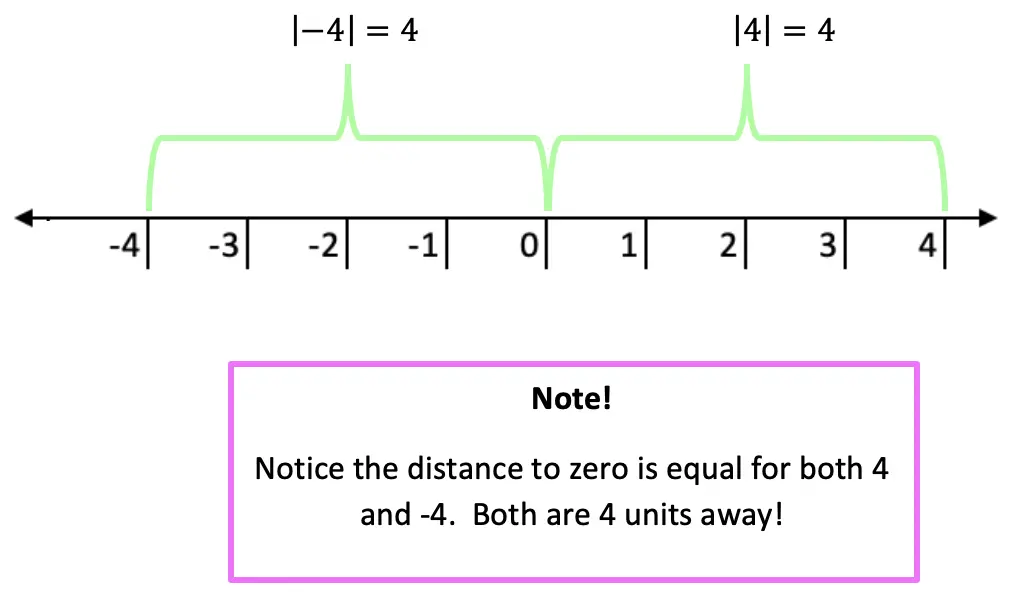Now let’s see how we can apply our knowledge of absolute value equations when there is a missing variable!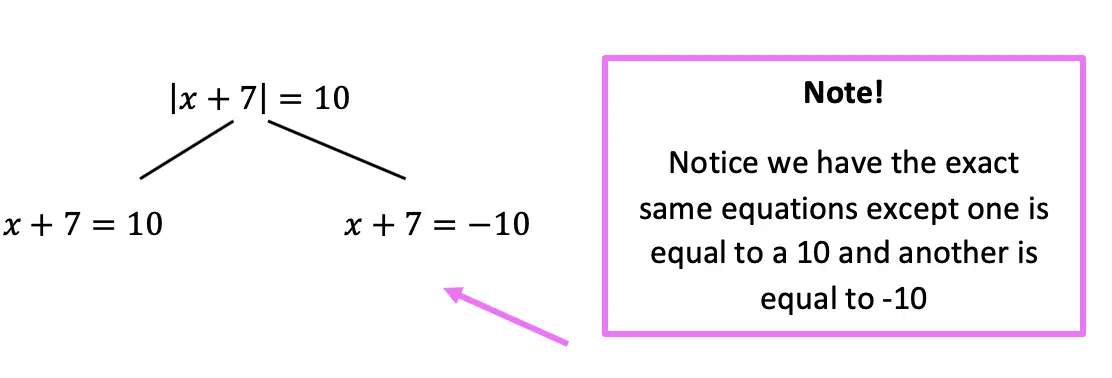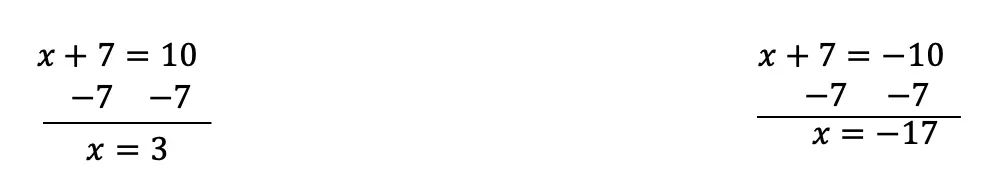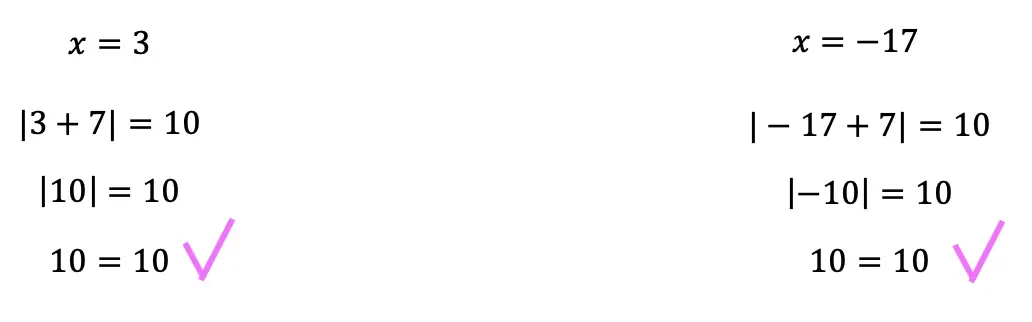Now let’s look at a slightly different example: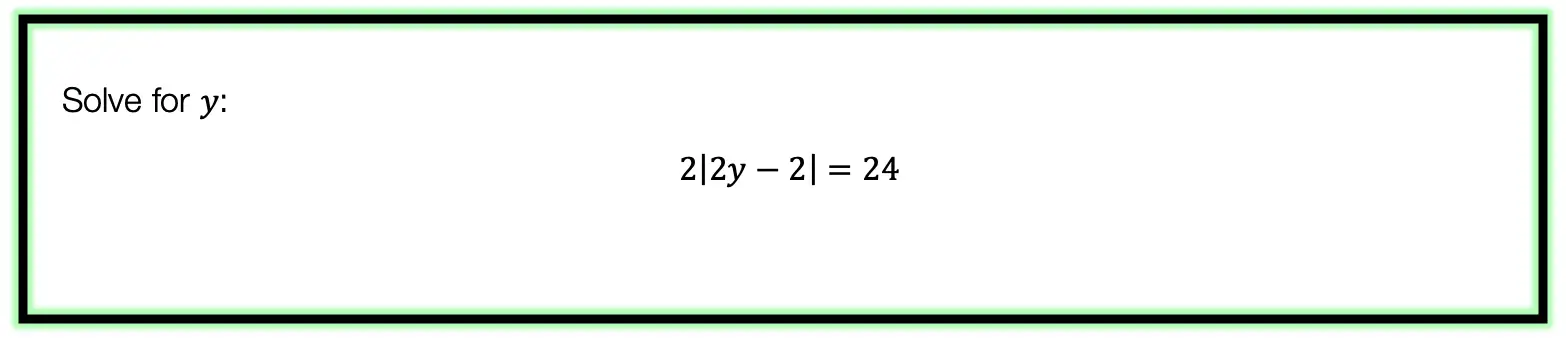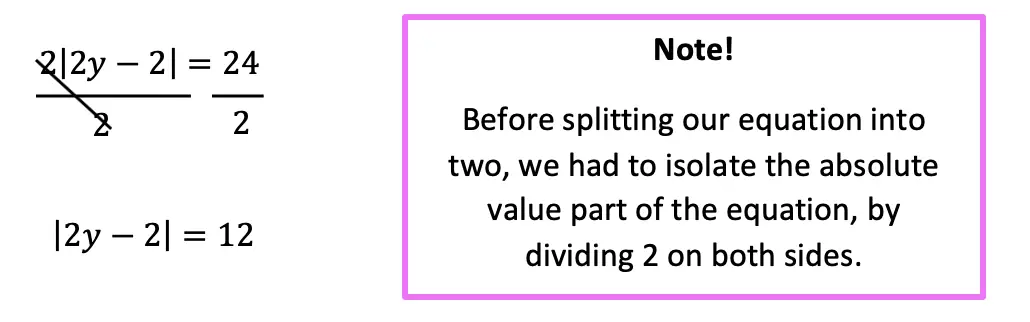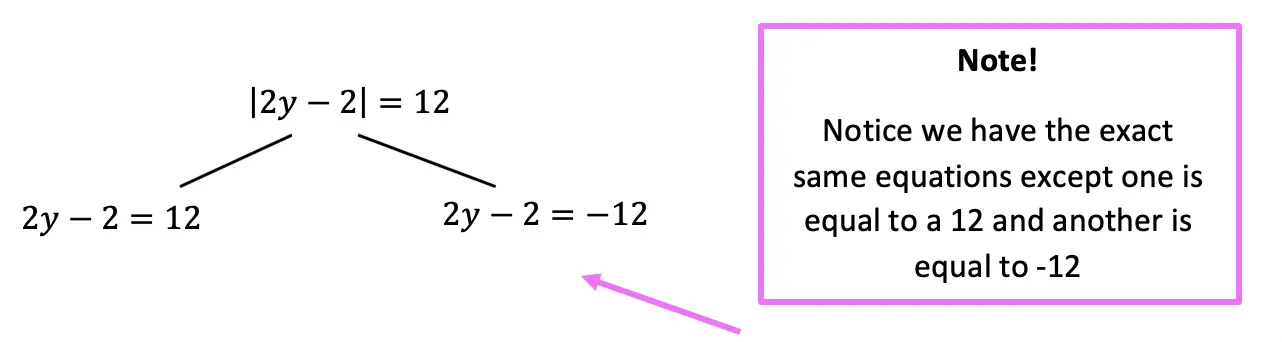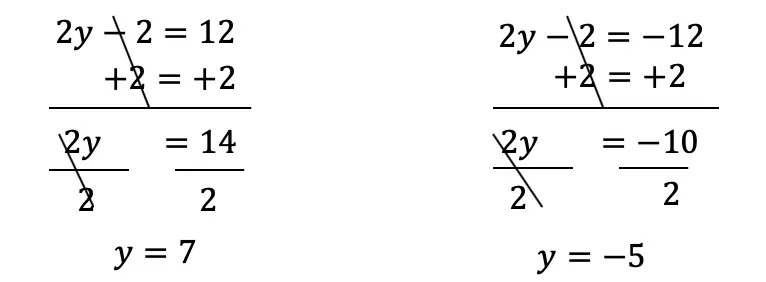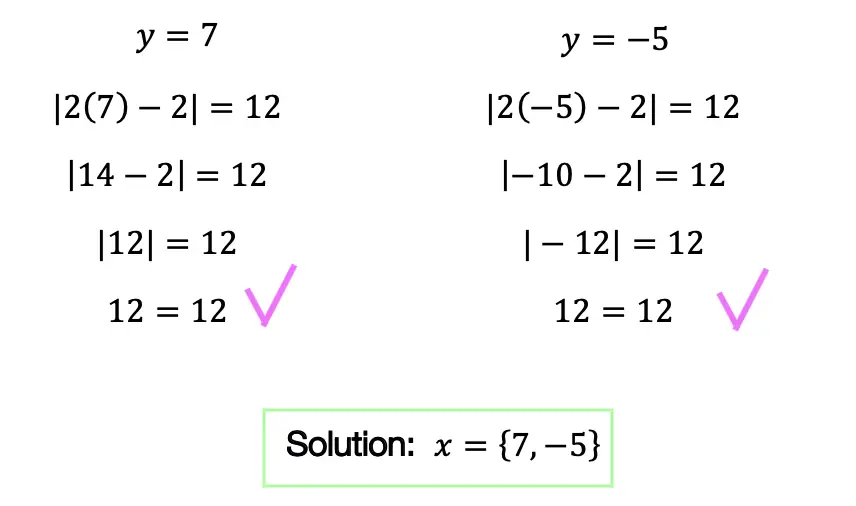Practice Questions: Given the following right triangles, find the missing lengths and side angles rounding to the nearest whole number.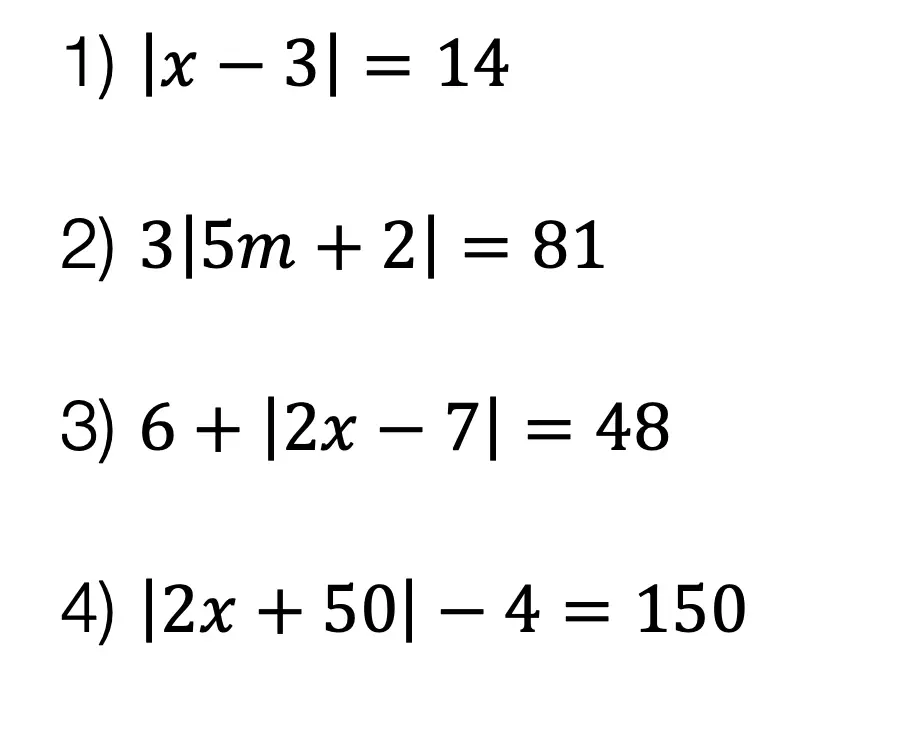Solutions: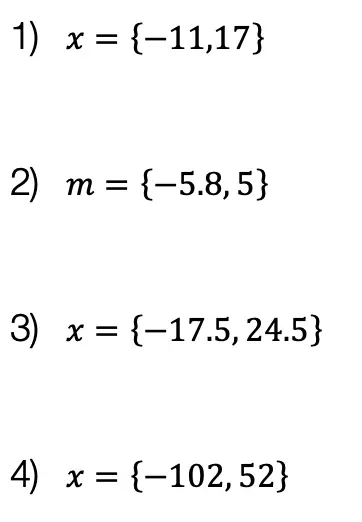Still got questions?  No problem! Check out the video the same examples outlined above. Happy calculating! 🙂

Also, if you’re looking for a review on combining like terms and the distributive property, check out this post here.

## How to use SOH CAH TOA: Geometry

Welcome back to Mathsux! This week, we’re going to go over how to find missing angles and side lengths of right triangles by using trigonometric ratios (sine, cosine, and tangent) (aka how to use SOH CAH TOA).  Woo hoo! These are the basics of right triangle trigonometry, and provides the base for mastering so many more interesting things in trigonometry! So, let’s get to it!

Also, if you have any questions about anything here, don’t hesitate to comment below or watch the video below. Also, don;r forget to subscribe to Math Sux for FREE math videos, lessons, and practice questions every week. Happy calculating! 🙂

## How to use SOH CAH TOA?

Trigonometric Ratios (more commonly known as Sine, Cosine, and Tangent) are ratios that naturally exist within a right triangle.  This means that the sides and angles of a right triangle are in proportion within itself.  It also means that if we are missing a side or an angle, based on what we’re given, we can probably find it!

Let’s take a look at what Sine, Cosine, and Tangent are all about!

Now let’s see how we can apply trig ratios when there is a missing side or angle in a right triangle!

Now for another type of question; using trig functions to find missing angles, let’s take a look:

Try the following Practice Questions on your own!

Solutions:

Still got questions?  No problem! Check out the video the same examples outlined above and happy calculating! 🙂

Need to brush up on special right triangles? Check out this posts on the 45 45 90 special triangles here!

## How to Solve Log Equations: Algebra 2/Trig.

Welcome to Mathsux! Today, we’re going to go over how to solve log equations, yay! But before we get into finding x, though, we need to go over what log equations are and why we use them in the first place…..just in case you were curious!

Also, if you have any questions about anything here, don’t hesitate to comment below or shoot me an email.  Happy calculating! 🙂

## What are Log Equations?

Logarithms are the inverses of exponential functions.  This means that when graphed, they are symmetrical along the line y=x.  Check it out below!

When on the same set of axis, notice how the functions are symmetrical over the line y=x:

We use logarithms to find the unknown values of exponents, such as the x value in the equation,.  This is a simple example, where we know the value of x is equal to 2,(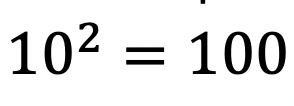). But what if it were to get more complicated?  That’s where logs come in!

## How to Solve Log Equations?

Logarithms follow a swooping pattern that allows us to write it in exponential form, let’s take a look at some Examples below:

But wait there’s more! Logs have a set of Rules that makes solving log equations a breeze!

We can use these rules to help us algebraically solve logarithmic equations, let’s look at an example that applies the Product Rule.

## Example:

Try the following practice questions on your own!

Practice Questions:

Solutions:

Still got questions?  No problem! Check out the video that goes over the same example outlined above.  And for more info. on logarithms check out this post that goes over a NYS Regent’s question here.  Subscribe below to get the latest FREE math videos, lessons, and practice questions from MathSux. Thanks for stopping by and happy calculating! 🙂

****Check out this Bonus Video on How to Change Log Bases****

## 4 Ways to Factor Trinomials: Algebra

Greeting math peeps and welcome to MathSux! In this post, we are going to go over 4 ways to Factor Trinomials and get the same answer, including, (1) Quadratic Formula (2) Product/Sum, (3) Completing the Square, and (4) Graphing on a Calculator.  If you’re looking for more don’t forget to check out the video and practice questions below.  Happy Calculating! 🙂

Also, if need a review on Factor by Grouping or Difference of Two Squares (DOTS) check out the hyperlinks here!

*If you haven’t done so, check out the video that goes over this exact problem, and don’t forget to subscribe!

We will take this step by step, showing 4 ways to factor trinomials, getting the same answer each and every time! Let’s get to it!

## 4 Ways to Factor Trinomials

____________________________________________________________________

## (2) Product/Sum: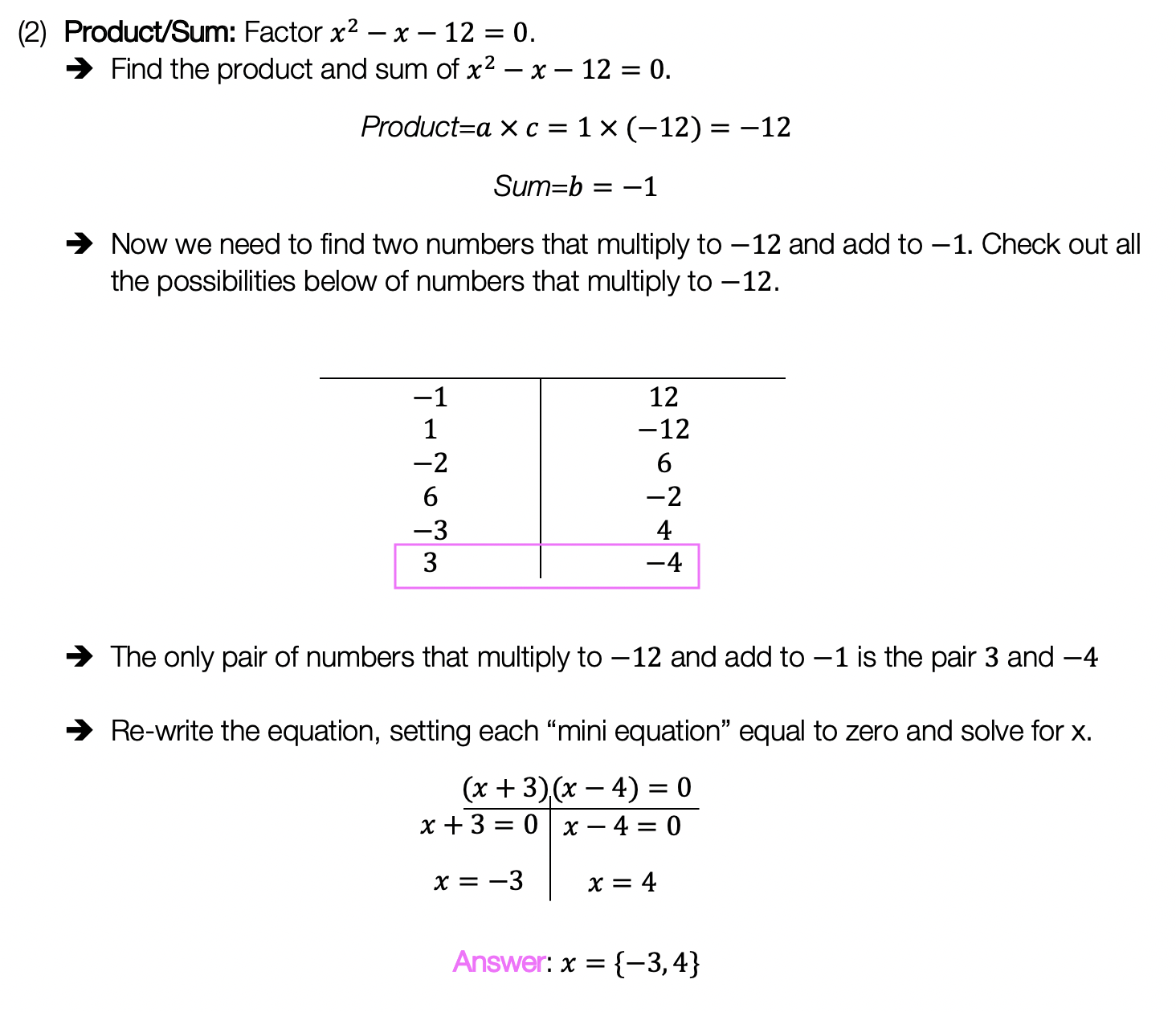____________________________________________________________________

## (3) Completing the Square: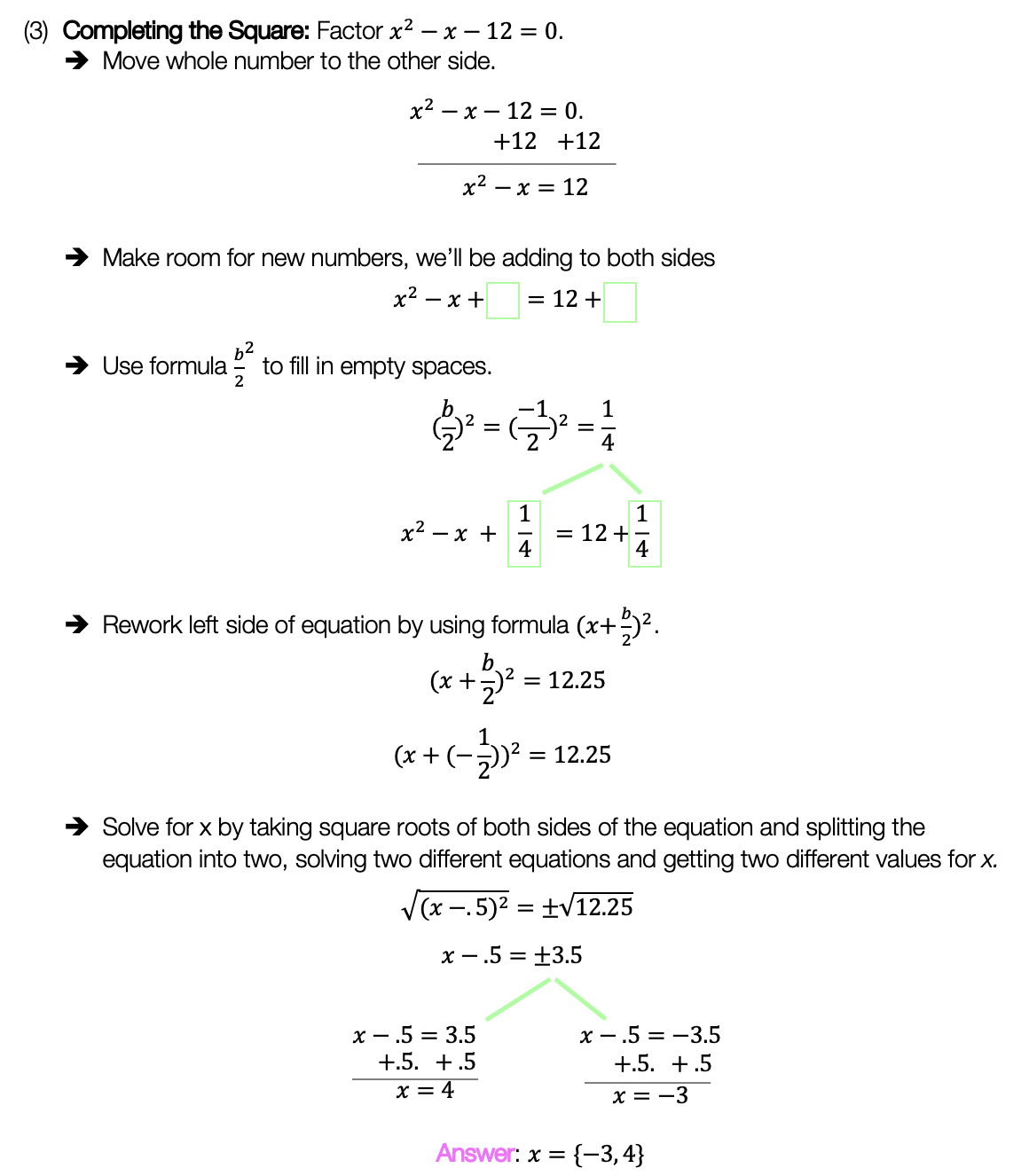____________________________________________________________________

## (4) Graph:

Choose the factoring method that works best for you and try the practice problems on your own below!

Practice Questions:

Solutions:

Want a review of all the different factoring methods out there?  Check out the ones left out here (DOTS and GCF) and happy calculating! 🙂

For even more ways to factor quadratic equations, check out How to factor by Grouping here! 🙂

Also, if you want more Mathsux?  Don’t forget to check out our Youtube channel and more below! If you have any questions, please don’t hesitate to comment below. Happy Calculating! 🙂

## Median of a Trapezoid Theorem: Geometry

Hi everyone and welcome to Math Sux! In this post, we are going to look at how to use and applythe median of a trapezoid theorem. Thankfully, it is not a scary formula, and one we can easily master with a dose of algebra. The only hard part remaining, is remembering this thing! Take a look below to see a step by step tutorial on how to use the median of a trapezoid theorem and check out the practice questions at the end of this post to truly master the topic. Happy calculating! 🙂

*If you haven’t done so, check out the video that goes over this exact problem, also please don’t forget to subscribe!

Step 1:  Let’s apply the Median of a Trapezoid Theorem to this question!  A little rusty?  No problem, check out the Theorem below.

Median of a Trapezoid Theorem: The median of a trapezoid is equal to the sum of both bases.Step 2: Now that we found the value of x , we can plug it back into the equation for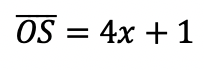median,  to find the value of median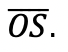Want more practice?  Your wish is my command! Check out the practice problems below:

Practice Questions:

1.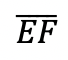is the median of trapezoid ABCDEF, find the value of the median, given the following:2.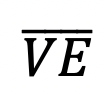is the median of trapezoid ACTIVE, find the value of the median, given the following:3.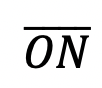is the median of  trapezoid DRAGON, find the value of the median, given the following:

4.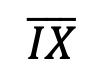is the median of trapezoid MATRIX, find the value of the median, given the following:

Solutions:

Need more of an explanation?  Check out the detailedand practice problems. Happy calculating! 🙂

## Solving Radical Equations: Algebra 2/Trig.

Today we’re back with Algebra 2, this time solving for radical equations!  Did you say “Radical Equations?” As in wild and crazy equations? No, not exactly, radicals in math are used to take the square root, cubed root, or whatever root of a number.

## Example #1:

Radicals are actually pretty cool because we can write them a couple of different ways and they all mean the same thing! Check it out below:Still not sure of their coolness? Let’s see what they look like with actual numbers:
Example: Solve the following algebraic equation below for the missing variable (aka, solve for x).Explanation:

How do I answer this question?

The question wants us to solve for x using our knowledge of radicals and algebra. You can also check out how to solve this question on Youtube here!

How do we do this?

Step 1: We start solving this radical equation like any other algebraic problem: by getting x alone. We can do this easily by subtracting 7 and then dividing out 5.

Step 2: Now, to get rid of that pesky radical, we need to square the entire radical.  Remember, whatever we do to one side of the equation, we must also do to the other side of the equation, therefore, we also square 14 on the other side of the equal sign. *This gets rid of our radical and allows us to solve for x algebraically as normal!

What happens when there is a cubed root though!?!?

When dividing polynomials with different value roots, raise the entire radical to that same power of root to cancel it out:Remember, we know radicals can also be written as fractions:

Therefore we also know that if we raise the entire radical expression to the same power of the root, the two exponents will cancel each other out:

## Example #2:

Want more practice? Try solving radical equations in the next few examples on your own.

## Solutions:

Looking to brush up on how to solve absolute value equations? Check out the post here! Did I miss anything?  Don’t let any questions go unchecked and let me know in the comments! Happy calculating! 🙂

Don’t forget to check out the latest free videos and posts with MathSux and subscribe!